# Absolute value - math problems

#### Number of problems found: 60

• Ferdinand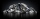Ferdinand went from place A to place B and from there to place C. A is 7.2 km from B and B is 11.7 km from C. Albert went from place A to place D and from there to place C. D is 9.1 km from A and C is 10.8 km from D. Who traveled more and by how much?
• Polygon - area coordinatesFind the perimeter and the area of the polygon with the given vertices. T (2,7), U (2,9), V (5,9), W (5,7)
• Distance two imaginary numbs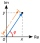Find the distance between two complex number: z1=(-8+i) and z2=(-1+i).
• A Cartesian framework1. In a Cartesian framework, the functions f and g we know that: the function (f) is defined by f (x) = 2x ^ 2, the function (g) is defined by g (x) = x + 3, the point (O) is the origin of the reference, point (C) is the point of intersection of the graph
• Modulus and argumentFind the mod z and argument z if z=i
• Three-digit numbers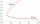How many three-digit numbers are not closer to 600 on the number axis than to 400?
• Space vectors 3DThe vectors u = (1; 3; -4), v = (0; 1; 1) are given. Find the size of these vectors, calculate the angle of the vectors, the distance between the vectors.
• Vectors abs sum diff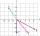The vectors a = (4,2), b = (- 2,1) are given. Calculate: a) |a+b|, b) |a|+|b|, c) |a-b|, d) |a|-|b|.
• Calculate 6Calculate the distance of a point A[0, 2] from a line passing through points B[9, 5] and C[1, -1].
• On a lineOn a line p : 3 x - 4 y - 3 = 0, determine the point C equidistant from points A[4, 4] and B[7, 1].
• Two parallel chordsIn a circle 70 cm in diameter, two parallel chords are drawn so that the center of the circle lies between the chords. Calculate the distance of these chords if one of them is 42 cm long and the second 56 cm.
• The modulus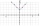Find the modulus of the complex number 2 + 5i
• Angle of the body diagonalsUsing vector dot product calculate the angle of the body diagonals of the cube.
• SupposeSuppose you know that the length of a line segment is 15, x2=6, y2=14 and x1= -3. Find the possible value of y1. Is there more than one possible answer? Why or why not?
• Two chordsIn a circle with radius r = 26 cm two parallel chords are drawn. One chord has a length t1 = 48 cm and the second has a length t2 = 20 cm, with the center lying between them. Calculate the distance of two chords.
• Temperature difference 2The temperature in London on new year’s day is -2 degree Celsius. The temperature in Moscow on the same day is -14 degree Celsius, what is the temperature difference between the two cities?
• LighthouseMarcel (point J) lies in the grass and sees the top of the tent (point T) and behind it the top of the lighthouse (P). | TT '| = 1.2m, | PP '| = 36m, | JT '| = 5m. Marcel lies 15 meters away from the sea (M). Calculate the lighthouse distance from the sea
• Cube rootFor 13, Sam wrote 2891 instead of the correct cube number. By how much was he wrong?
• Coordinates of square verticesThe ABCD square has the center S [−3, −2] and the vertex A [1, −3]. Find the coordinates of the other vertices of the square.
• Balloon and bridgeFrom the balloon, which is 92 m above the bridge, one end of the bridge is seen at a depth angle of 37° and the second end at depth angle 30° 30 '. Calculate the length of the bridge.

Do you have an exciting math question or word problem that you can't solve? Ask a question or post a math problem, and we can try to solve it.

We will send a solution to your e-mail address. Solved examples are also published here. Please enter the e-mail correctly and check whether you don't have a full mailbox.

Please do not submit problems from current active competitions such as Mathematical Olympiad, correspondence seminars etc...